Equivalent Fractions

Chapter 7 Class 6 Fractions
Concept wise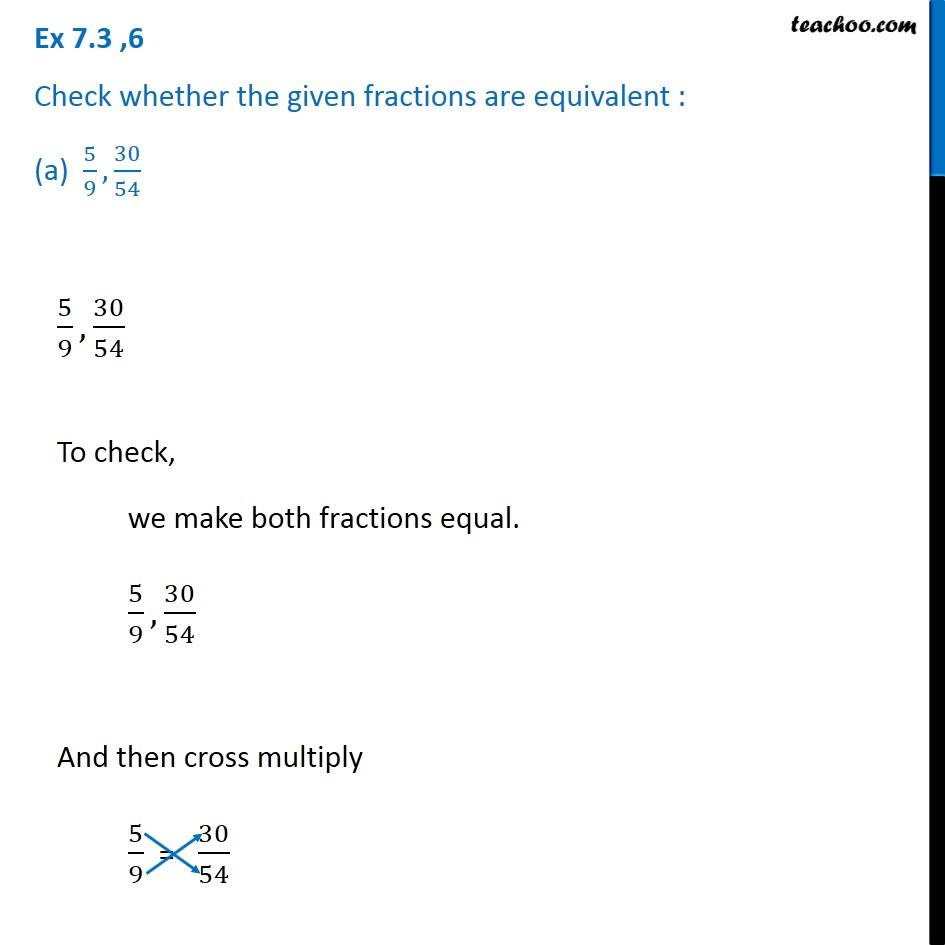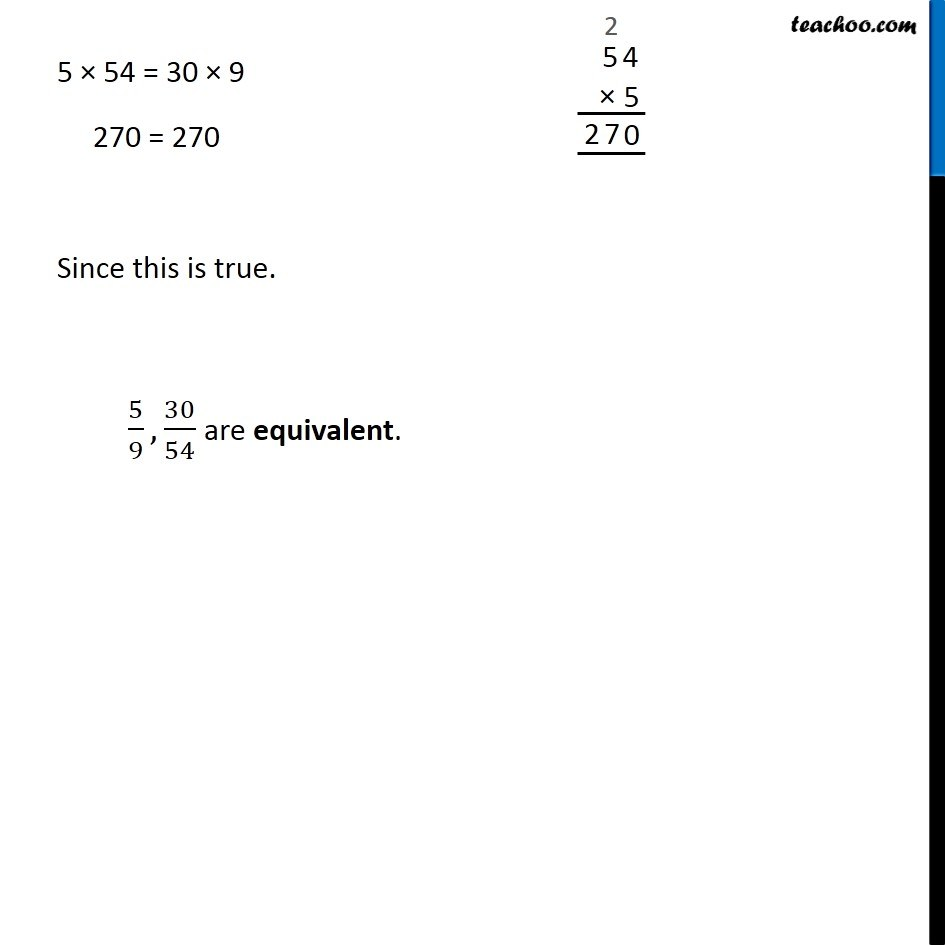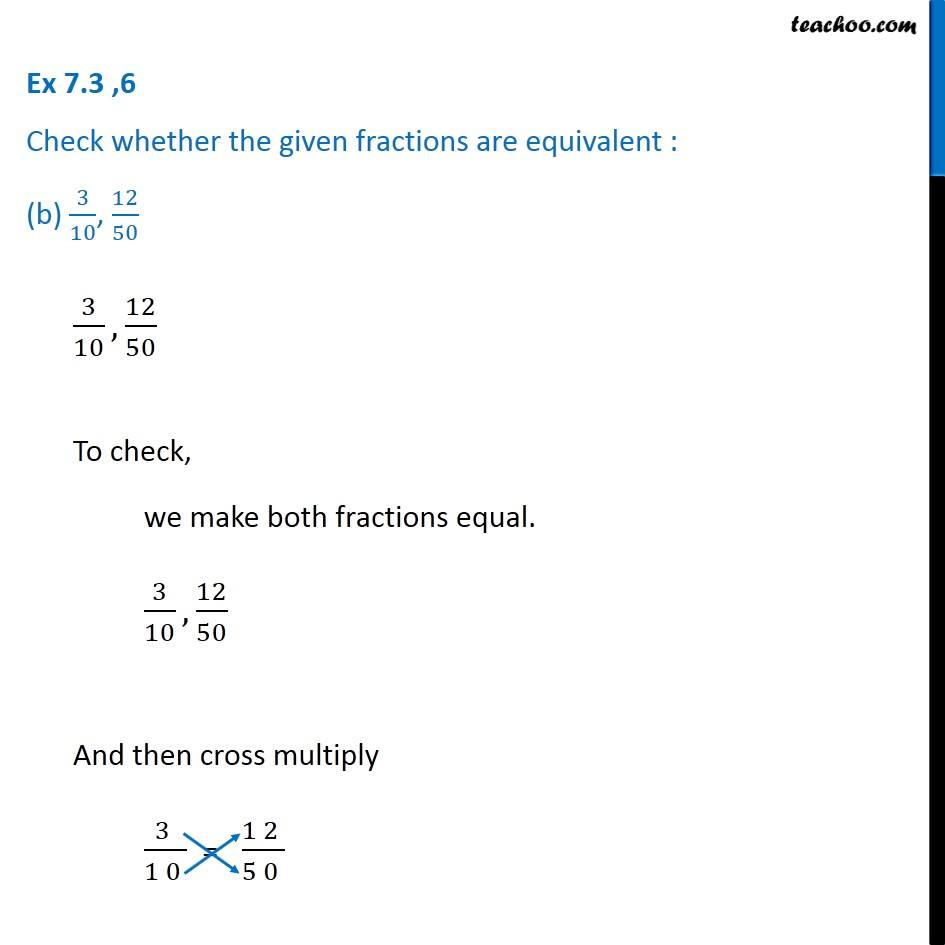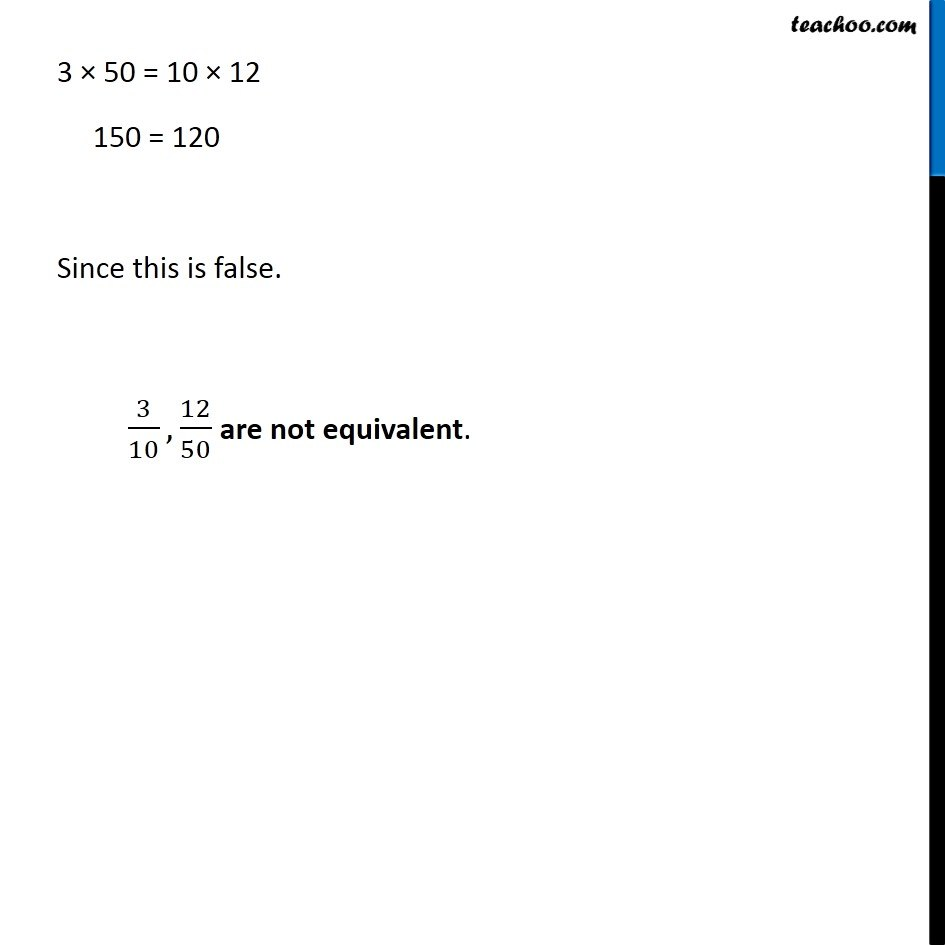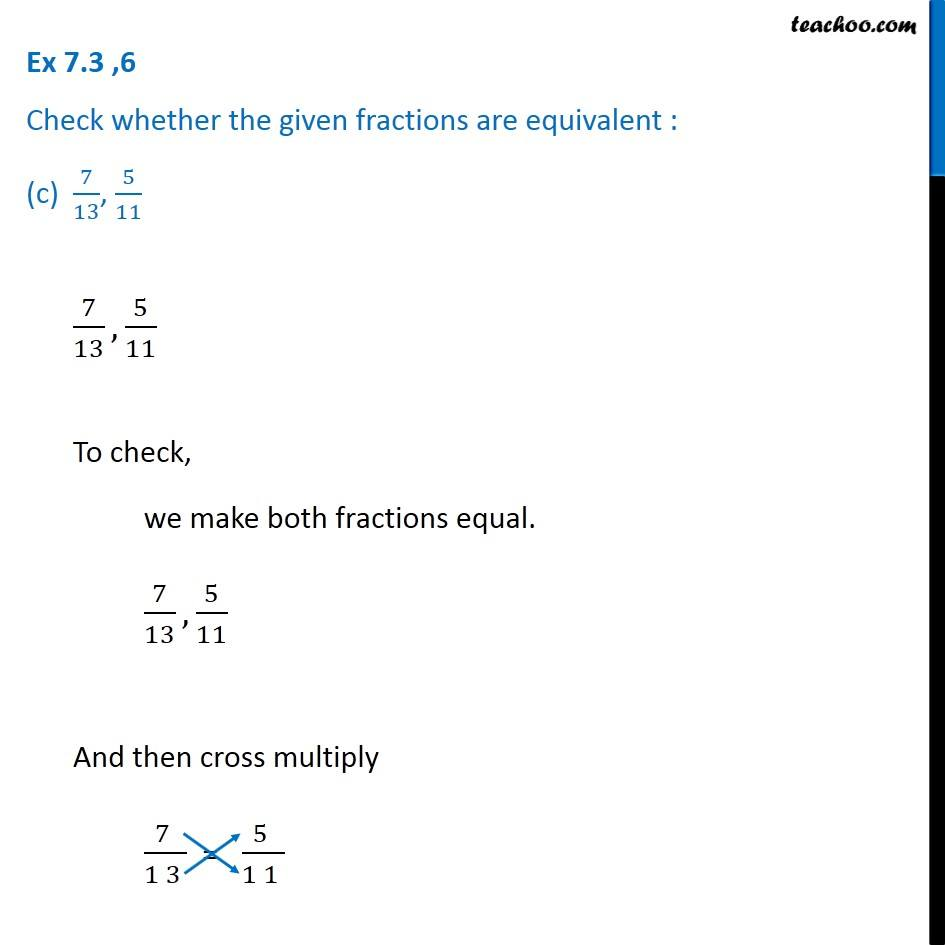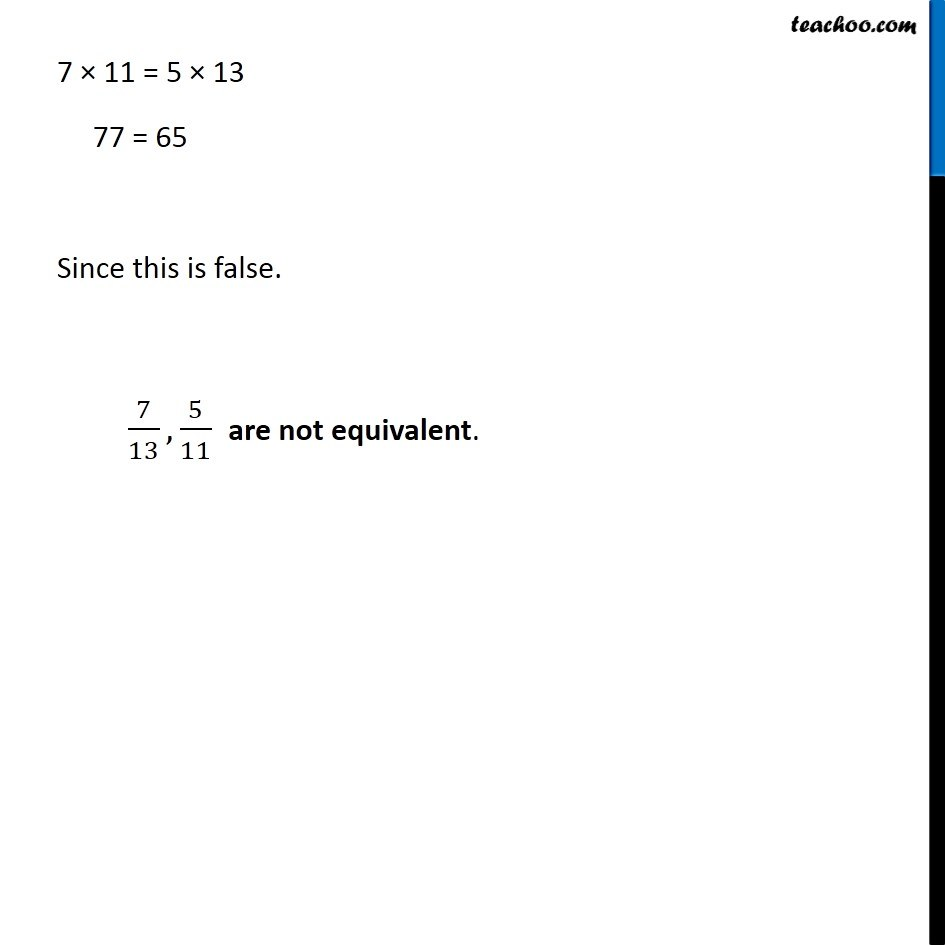Introducing your new favourite teacher - Teachoo Black, at only ₹83 per month

### Transcript

Ex 7.3 ,6 Check whether the given fractions are equivalent : (a) 5/9, 30/545/9, 30/54 To check, we make both fractions equal. 5/9, 30/54 And then cross multiply 5/9 = 30/54 5 × 54 = 30 × 9 270 = 270 Since this is true. 5/9, 30/54 are equivalent. Ex 7.3 ,6 Check whether the given fractions are equivalent : (b) 3/10, 12/503/10, 12/50 To check, we make both fractions equal. 3/10, 12/50 And then cross multiply 3/10 = 12/50 3 × 50 = 10 × 12 150 = 120 Since this is false. 3/10, 12/50 are not equivalent. Ex 7.3 ,6 Check whether the given fractions are equivalent : (c) 7/13, 5/117/13, 5/11 To check, we make both fractions equal. 7/13, 5/11 And then cross multiply 7/13 = 5/11 7 × 11 = 5 × 13 77 = 65 Since this is false. 7/13, 5/11 are not equivalent.